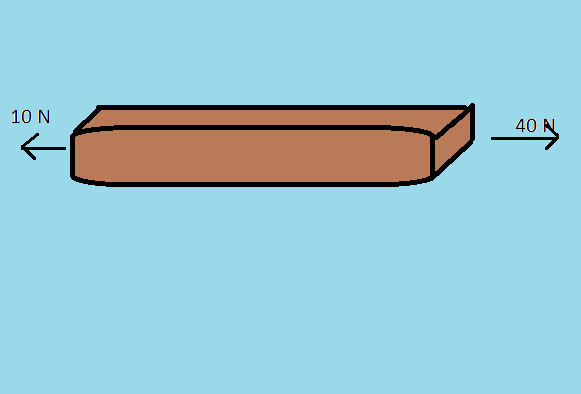# Without calculusImagine a rod made of a material of youngs modulus Y and length l, area of cross section A , in Space far away from all gravitational fields

It is subject to 2 forces one 40N and other 10N acting on opposite faces in opposite directions as shown,

Find the net elongation of rod

find 100a

NOTE

1) Any one can solve using calculus, try to do it without using calculus

2) Remembering a result or formula and solving it is not the same as a new method, in short- Try to Avoid using calculus based results for this problem

-DETAILS AND ASSUMPTIONS

1) L=1

2) A=5

3) Y=5

All in SI units

×